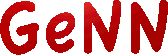GeNN  4.7.1 GPU enhanced Neuronal Networks (GeNN)
Python interface (PyGeNN)

As well as being able to build GeNN models and user code directly from C++, you can also access all GeNN features from Python. The `pygenn.GeNNModel` class provides a thin wrapper around `ModelSpec` as well as providing support for loading and running simulations; and accessing their state. `SynapseGroup`, `NeuronGroup` and `CurrentSource` are similarly wrapped by the `pygenn.SynapseGroup`, `pygenn.NeuronGroup` and `pygenn.CurrentSource` classes respectively.

Full installation instructions can be found in Installing PyGeNN. The following example shows how PyGeNN can be easily interfaced with standard Python packages such as numpy and matplotlib to plot 4 different Izhikevich neuron regimes:

import numpy as np
import matplotlib.pyplot as plt
from pygenn.genn_model import GeNNModel
# Create a single-precision GeNN model
model = GeNNModel("float", "pygenn")
# Set simulation timestep to 0.1ms
model.dT = 0.1
# Initialise IzhikevichVariable parameters - arrays will be automatically uploaded
izk_init = {"V": -65.0,
"U": -20.0,
"a": [0.02, 0.1, 0.02, 0.02],
"b": [0.2, 0.2, 0.2, 0.2],
"c": [-65.0, -65.0, -50.0, -55.0],
"d": [8.0, 2.0, 2.0, 4.0]}
# Add neuron populations and current source to model
pop = model.add_neuron_population("Neurons", 4, "IzhikevichVariable", {}, izk_init)
model.add_current_source("CurrentSource", "DC", "Neurons", {"amp": 10.0}, {})
model.build()
# Create a numpy view to efficiently access the membrane voltage from Python
voltage_view = pop.vars["V"].view
# Simulate
v = None
while model.t < 200.0:
model.step_time()
model.pull_state_from_device("Neurons")
v = np.copy(voltage_view) if v is None else np.vstack((v, voltage_view))
# Create plot
figure, axes = plt.subplots(4, sharex=True)
# Plot voltages
for i, t in enumerate(["RS", "FS", "CH", "IB"]):
axes[i].set_title(t)
axes[i].set_ylabel("V [mV]")
axes[i].plot(np.arange(0.0, 200.0, 0.1), v[:,i])
axes[-1].set_xlabel("Time [ms]")
# Show plot
plt.show()